# Modeling Amplifiers as Analog Filters Increases SPICE Simulation Speed

### Introduction

Simulation models for amplifiers are typically implemented with resistors, capacitors, transistors, diodes, dependent and independent sources, and other analog components. An alternative approach uses a second-order approximation of the amplifier’s behavior (Laplace transform), speeding up the simulation and reducing the simulation code to as little as three lines.

With high-bandwidth amplifiers, however, time-domain simulations using s-domain transfer functions can be very slow, as the simulator must first calculate the inverse transform and then convolve it with the input signal. The higher the bandwidth, the higher the sampling frequency required to determine the time-domain function. This results in increasingly difficult convolution calculations, slowing down the time-domain simulations.

This article presents a further refinement, synthesizing the second-order approximation as an analog filter rather than as an s-domain transfer function to provide much faster time-domain simulations, especially for higher bandwidth amplifiers.

### Second-Order Transfer Functions

The second-order transfer function can be implemented using the Sallen-Key filter topology, which requires two resistors, two capacitors, and a voltage-controlled current source for an amplifier simulation model; or the multiple feedback (MFB) filter topology, which requires three resistors, two capacitors, and a voltage-controlled current source. Both topologies should give the same results, but the Sallen-Key topology is simpler to design, while the MFB topology has better high-frequency response and might be better for programmable-gain amplifiers, as it is easier to switch in different resistor values.

We can begin the process by modeling the frequency and transient response of an amplifier with the following standard form for the second-order approximation:

Conversions to Sallen-Key and multiple feedback topologies are shown in Figure 1.Figure 1 . Filter topologies.

The natural undamped frequency of the amplifier, ωn, is equal to the corner frequency of the filter, ωc, and the damping ratio of the amplifier, ζ, is equal to ½ times the reciprocal the quality factor of the filter, Q. For a two-pole filter, Q indicates the radial distance of the poles from the jω-axis, with higher values of Q indicating that the poles are closer to the jω-axis. With amplifiers, larger damping ratios result in lower peaking. These relationships serve as useful equivalencies between the s-domain (s = jω) transfer function and the analog filter circuit.

### Design Example: Gain-of-5 Amplifier

The design consists of three major steps: first, measure the amplifier’s overshoot (Mp) and settling time (ts). Second, using these measurements, calculate the second-order approximation of the amplifier’s transfer function. Third, convert the transfer function to the analog filter topology to produce the amplifier’s SPICE model.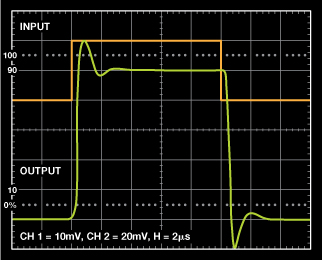Figure 2. Gain-of-5 amplifier.

As an example, a gain-of-5 amplifier will be simulated using both Sallen-Key and MFB topologies. From Figure 2, the overshoot (Mp) is approximately 22%, and the settling time to 2% is approximately 2.18 μs. The damping ratio, ζ, is calculated as

Rearranging terms to solve for ζ gives

Next, calculate the natural undamped frequency in radians per second using the settling time.

For a step input, the s2 and s terms in the denominator of the transfer function (in radians per second) are calculated from

and

The unity-gain transfer function then becomes

The final transfer function for a gain-of-5 amplifier is obtained by multiplying the step function by 5:

The following netlist simulates the Laplace transform for the transfer function of the gain-of-5 amplifier. Before converting to a filter topology, it’s good to run simulations to verify the Laplace transform, adjusting the bandwidth as needed by making the settling time larger or smaller.

***GAIN_OF_5 TRANSFER FUNCTION***

.SUBCKT SECOND_ORDER +IN –IN OUT

E1 OUT 0 LAPLACE {V(+IN) – V(–IN)} = {89.371E12 / (S^2 + 3.670E6*S + 17.874E12)}

.END

Figure 3 shows the simulation results in the time domain. Figure 4 shows the results in the frequency domain.Figure 3. Gain-of-5 amplifier: time domain simulation results.Figure 4. Gain-of-5 amplifier: frequency domain simulation results.

The peaking in the pulse response makes it easy to maintain a constant damping ratio while varying the settling time to modify the bandwidth. This changes the angle of the complex-conjugate pole pair with respect to the real axis in an amount equal to the arccosine of the damping ratio, as shown in Figure 5. Decreasing the settling time increases the bandwidth; and increasing the settling time decreases the bandwidth. Peaking and gain will not be affected as long as the damping ratio is kept constant and adjustments are made only to the settling time, as shown in Figure 6.Figure 5. Complex conjugate pole-pair of the gain-of-5 transfer function.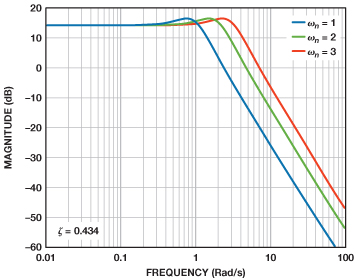Figure 6. Bandwidth due to settling time adjustment.

Once the transfer function matches the characteristics of the actual amplifier, it is ready to be converted to a filter topology. This example will use both Sallen-Key and MFB topologies.

First, use the canonical form for the unity-gain Sallen-Key topology to convert the transfer function into resistor and capacitor values.

From the s-term, C1 can be found from

Choose convenient resistor values, such as 10 kΩ, for R1 and R2, and calculate C1.

Use the relationship for the corner frequency to solve for C2.

The resulting netlist follows, and the Sallen-Key circuit is illustrated in Figure 7. E1 multiplies the step function to obtain a gain of 5. Ro provides an output impedance of 2 Ω. G1 is a VCCS with a gain of 120 dB. E2 is the differential input block. The frequency vs. gain simulation was identical to the simulation using the Laplace transform.

.SUBCKT SALLEN_KEY +IN –IN OUT

R1 1 4 10E3

R2 5 1 10E3

C2 5 0 10.27E–12

C1 2 1 54.5E–12

G1 0 2 5 2 1E6

E2 4 0 +IN –IN 1

E1 3 0 2 0 5

RO OUT 3 2

.END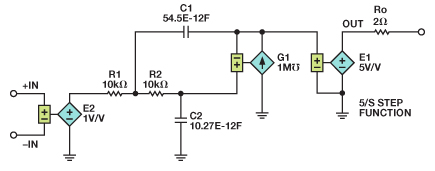Figure 7. Simulation circuit for gain-of-5 amplifier using Sallen-Key filter.

Next, use the standard form for the MFB topology to convert the transfer function into resistor and capacitor values.

Begin the transformation by calculating R2. To do this, the transfer function can be restated in this more generic form

Set C1 = 10 nF. Next, choose C2 such that the quantity under the radical is positive. For convenience, C2 was chosen as 10 pF. Substituting the known values of C2 = 10 pF, a1 = 3.67E6, K = 5, and a0 = 17.86E12 gives the value for R2:

R1 can easily be found as R2/K = R2/5 = 33. From the standard polynomial coefficients, solve for R3. Substituting known values for a0, R2, and C2 gives

Finally, to verify that the component ratios are correct, C1 should equal 10 nF after substituting known values for a0, R2, R3, gain K, and C2 (from the s term).

Now that the component values are solved, substitute back into the equations to verify that the polynomial coefficients are mathematically correct. A spreadsheet calculator is an easy way to do this. The component values shown provide practical values for use in the final SPICE model. In practice, ensure that the minimum capacitor value does not fall below 10 pF.

The netlist for the gain-of-5 amplifier follows and the model is shown in Figure 8. G1 is a VCCS (voltage-controlled current source) with an open-loop gain of 120 dB. Note that the component count is much lower than would otherwise be required with transistors, capacitors, diodes, and dependent sources.

.SUBCKT MFB +IN –IN OUT

***VCCS – 120 dB OPEN_LOOP_GAIN***

G1 0 7 0 6 1E6

R1 4 3 330

R3 6 4 34K

C2 7 6 1P

C1 0 4 1N

R2 7 4 1.65K

E2 3 0 +IN –IN 1

E1 9 0 7 0 –1

***OUTPUT_IMPEDANCE RO = 2 Ω***

RO OUT 9 2

.ENDFigure 8. Simulation circuit for gain-of-5 amplifier using MFB filter.

### Design Example: Gain-of-10 Amplifier

As a second example, consider the pulse response of a gain-of-10 amplifier without overshoot, as shown in Figure 9. The settling time is approximately 7 μs. Since there is no overshoot, the pulse response can be approximated as being critically damped, with ζ ≈ 0.935 (Mp = 0.025%).Figure 9. Gain-of-10 amplifier with no overshoot.

With no overshoot, it is convenient to maintain a constant settling time and adjust the damping ratio to simulate the correct bandwidth and peaking. Figure 10 shows how the poles move as the damping ratio is varied while maintaining a constant settling time. Figure 11 shows the change in frequency response.Figure 10. Pole locations for different damping ratios with constant setting time.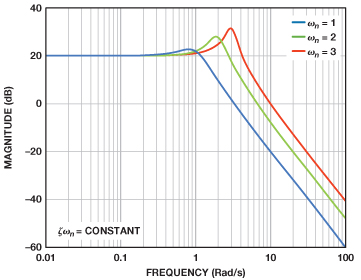Figure 11. Frequency response for different damping ratios with constant setting time.

***AD8208 PREAMPLIFIER_TRANSFER_FUNCTION (GAIN = 20 dB)***

.SUBCKT PREAMPLIFIER_GAIN_10 +IN –IN OUT

E1 OUT 0 LAPLACE {V(+IN)–V(–IN)} = {3.734E12 / (S^2 + 1.143E6*S + 373.379E9)}

.END

To find resistor and capacitor values for the unity gain Sallen-Key topology, choose R1 = R2 = 10 kΩ as before. Calculate the capacitor values with the same method used in the gain-of-5 amplifier example:

The netlist follows and the Sallen-Key simulation circuit model is shown in Figure 12. E2, a gain-of-10 block, is placed at the output stage along with a 2-Ω output impedance. E2 multiplies the unity gain transfer function by 10. Both Laplace and Sallen-Key netlists produced identical simulations, as shown in Figure 13.

***AD8208 PREAMPLIFIER_TRANSFER_FUNCTION (GAIN = 20 dB)***

.SUBCKT AMPLIFIER_GAIN_10_SALLEN_KEY +IN –IN OUT

R1 1 4 10E3

R2 5 1 10E3

C2 5 0 153E–12

C1 2 1 175E–12

G1 0 2 5 2 1E6

E2 4 0 +IN –IN 10

E1 3 0 2 0 1

RO OUT 3 2

.ENDFigure 12. Simulation circuit for gain-of-10 amplifier using Sallen-Key filter.Figure 13. Frequency domain simulation of gain-of-10 amplifier using Sallen-Key filter.

A similar derivation can be done using the MFB topology. The netlist follows, and the simulation model is shown in Figure 14.

***AD8208 PREAMPLIFIER_TRANSFER_FUNCTION (GAIN = 20 dB)***

.SUBCKT 8208_MFB +IN –IN OUT

***G1 = VCCS WITH 120 dB OPEN_LOOP_GAIN***

G1 0 7 0 6 1E6

R1 4 3 994.7

R2 7 4 9.95K

R3 6 4 26.93K

C1 0 4 1N

C2 7 6 10P

EIN_STAGE 3 0 +IN –IN 1

***E2 = OUTPUT BUFFER***

E2 9 0 7 0 1

***OUTPUT RESISTANCE = 2 Ω***

RO OUT 9 2

.ENDFigure 14. Simulation circuit for gain-of-10 amplifier using MFB filter.

### Conclusion

SPICE models constructed with analog components will provide much faster time-domain simulations for higher bandwidth amplifiers as compared to those of s-domain (Laplace transform) transfer functions. The Sallen-Key and MFB low-pass filter topologies provide a method for converting s-domain transfer functions into resistors, capacitors, and voltage-controlled-current-sources.

Non-ideal operation of the MFB topology results from C1 and C2 behaving as shorts at high frequencies relative to the impedance of resistors R1, R2, and R3. Similarly, non-ideal operation of the Sallen-Key topology results from C1 and C2 behaving as shorts at high frequencies relative to the impedance of resistors R1 and R2. A comparison of the two topologies is shown in Figure 15.

Existing circuits commonly used for CMRR, PSRR, offset voltage, supply current, spectral noise, input/output limiting, and other parameters can be combined with the model, as shown in Figure 16.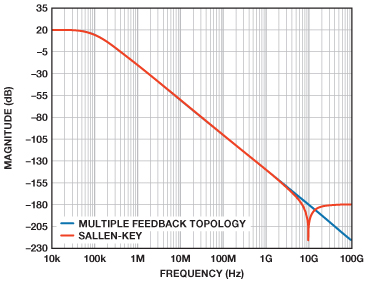Figure 15. Bode plots of Sallen-Key and MFB topologies.Figure 16. Complete SPICE amplifier model including error terms.

### Ссылки

Karpaty, David. “Create Spice Amplifier Models Using Second-Order Approximations.Electronic Design, September 22, 2010.